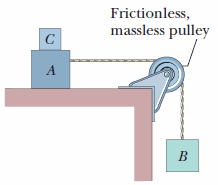# HW22#7 – Blocks A and B have weights of 54 N and 30 N. Determine the minimum weight of block C …

In the figure, blocks A and B have weights of 54 N and 30 N, respectively.(a) Determine the minimum weight of block C to keep A from sliding, if μs between A and the table is 0.20.

(b) Block C suddenly is lifted off A. What is the acceleration of block A, if μk between A and the table is 0.15?